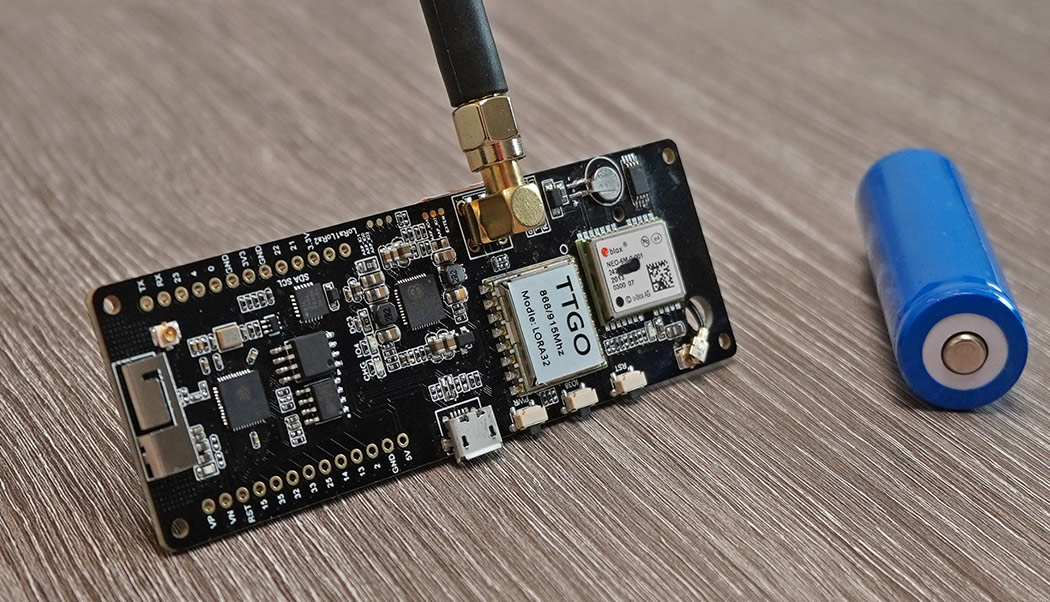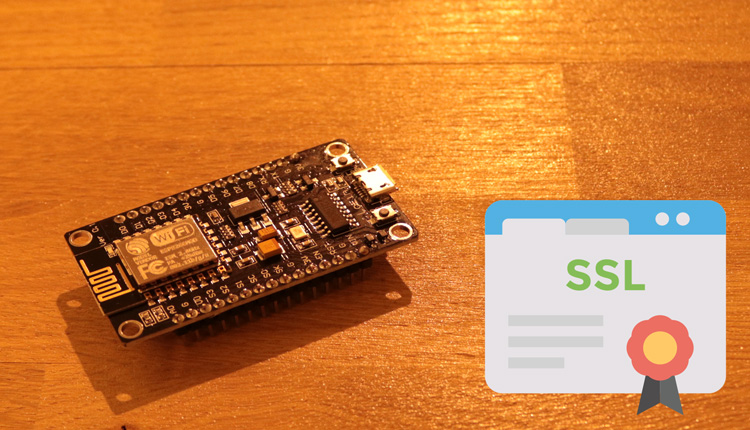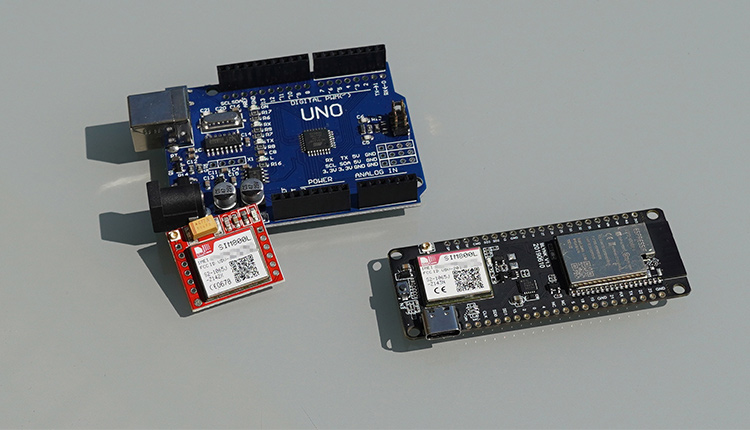## AEQ-WEB | Blog### ESP32 TTGO GPS NEO6

The TTGO GPS board is a very powerful development board and has besides the UBLOX NEO-6M GPS module also a LoRa module built in. On the backside there is a battery holder for a 18650 cell (Li-Ion battery), which allows a portable operation. In addition, the battery can also be charged directly via USB. A common ESP32 is used as the main microcontroller. By combining LoRa and GPS, this board can be used with some software to build an awesome and very cheap LoRaWAN GPS tracker. This article is about the hardware overview and startup of the GPS module as well as the conversion of the coordinates from degrees and minutes to decimal degrees (LAT, LON).

Related articles to this project:

#### The Lilygo Board

In contrast to known TTGO boards, this board is significantly larger. The reason for this is not only the large battery socket, but also many components that are installed on this board. Beside numerous ICs for SRAM, EEPROM, UART there is a Li-Ion Power Controller (AXP192) on this board. This controller is used to charge the battery and control the power supply of components like ESP32, GPS and LoRa. With the power management the energy consumption for the LoRa Node can be optimized so that a battery operation from months up to years is possible. There is also a user button and a freely programmable LED on the board. The range of LoRa is satisfactory and can be improved with another antenna.

Attention: There are several versions of this board with different pin assignments. The pin mapping may need to be adjusted before uploading.

#### The GPS module

The Ublox NEO-6M GPS module is installed as GPS receiver. When delivered, the module is configured to output the current position via a serial interface in NMEA-0183 format. In addition to the coordinates, you get a lot of other information, such as the current time, date, number of active satellites or signal quality, or even the height above sea level in meters. There is also a red LED on the GPS module, which starts flashing when GPS is fixed. UBLOX provides a comprehensive software, with which all parameters and settings can be changed via UART. Normally this is not necessary, because the preset configuration is sufficient. In the delivery state, the set baud rate is 9600. The update interval is approximately one second.

#### Read GPS data from serial

Several times per second current position data in different NMEA 0183 data sets are sent via the serial interface. Therefore a serial interface (Hardware Serial) is opened at the ESP32, which receives the data from the GPS module. Attention: The pinout of the GPS module may vary depending on TTGO model and version. You can find this information directly on the PCB. The following code example forwards received data sets to the Serial Monitor.

```//More information at: https://www.aeq-web.com/

#define SERIAL1_RX 34 // GPS_TX -> 34
#define SERIAL1_TX 12 // 12 -> GPS_RX

void setup(){
Serial.begin(115200);
Serial.println("TTGO GPS TEST");
delay(2000);
Serial1.begin(9600, SERIAL_8N1, SERIAL1_RX, SERIAL1_TX);
}

void loop() {
if (Serial1.available()) {
Serial.write(Serial1.read());
Serial1.println();
}
}
```

The following screenshot shows the output of the NMEA data records in the Serial Monitor during GPS receive. The red marked data record starting with "\$GPGAA,..." contains besides date, time and altitude also the position data in gard and minutes as well as the orientation (north, south & west, east). The individual parameters are subsequently generated from this data set.

#### Split NMEA-0183 Data Sentence

Several times per second different NMEA data sets are sent from the module to the ESP32. In the single data sets there are different parameters. It is sufficient if the line beginning with "\$GPGGA,.." is used for the position determination. Each parameter in this line is terminated with a comma (","). It is therefore necessary to develop a software that filters out the individual parameters from the data sets. The following graphic shows the breakdown of important values of a single NMEA data set:

The following sample code fetches the individual parameters from the GPGGA-Sentence:

```//More information at: https://www.aeq-web.com/

#define SERIAL1_RX 34 // GPS_TX -> 34
#define SERIAL1_TX 12 // 12 -> GPS_RX
String read_sentence;

void setup() {
Serial.begin(115200);
Serial.println("TTGO GPS TEST");
delay(2000);
Serial1.begin(9600, SERIAL_8N1, SERIAL1_RX, SERIAL1_TX);
}

void loop() {
read_sentence = Serial1.readStringUntil(13); //13 = return (ASCII)
read_sentence.trim();

if (read_sentence.startsWith("\$GPGGA")) {
String gps_lat = sentence_sep(read_sentence, 2); //Latitude in degrees & minutes
String gps_lon = sentence_sep(read_sentence, 4); //Longitude in degrees & minutes
String gps_sat = sentence_sep(read_sentence, 7);
String gps_hgt = sentence_sep(read_sentence, 9);
String gps_lat_o = sentence_sep(read_sentence, 3); //Orientation (N or S)
String gps_lon_o = sentence_sep(read_sentence, 5); //Orientation (E or W)

Serial.print("LAT: ");
Serial.print(gps_lat);
Serial.print(" LON: ");
Serial.print(gps_lon);
Serial.print(" HEIGHT: ");
Serial.print(gps_hgt);
Serial.print(" SAT: ");
Serial.println(gps_sat);
}
}

String sentence_sep(String input, int index) {
int finder = 0;
int strIndex[] = { 0, -1 };
int maxIndex = input.length() - 1;

for (int i = 0; i <= maxIndex && finder <= index; i++) {
if (input.charAt(i) == ',' || i == maxIndex) {  //',' = separator
finder++;
strIndex = strIndex + 1;
strIndex = (i == maxIndex) ? i + 1 : i;
}
}

return finder > index ? input.substring(strIndex, strIndex) : "";
}
```

Each NMEA line is terminated with a return character. Therefore in the first line of the loop each data record is read in until the return, which corresponds to the number 13 in ASCII code. At the same time this line is converted into a string and buffered. Then the previously buffered line is read in and checked if it starts with "\$GPGAA". If this is the case, it is the correct line with the desired parameters. Then the individual parameters are copied out of the line. This is done by the string function "sentence_sep()". As can be seen in the graphic above, the latitude, for example, is at the second position (starting from zero). The separator function reads the text between the second and third decimal point and returns all values in this range via a return. Then a string with the value is created and returned to the serial monitor. As can be seen in the graphic above, the latitude, for example, is at the second position (starting from zero). The separator function reads the text between the second and third decimal point and returns all values in this range via a return. Then a string with the respective value is created and printed out via the serial monitor.

Note: In general, numerical values should not be stored as strings. So that decimal places and possibly existing zeros before a number do not disappear, a data type optimization is omitted for this moment.

#### Conversion of the coordinates

The GPS module outputs the coordinates in degrees and minutes(GM), as well as the orientation. In most systems and applications like Google Maps, coordinates are given in decimal degrees (LAT, LON). The following graphic shows how coordinates are converted from GM to decimal degrees:

The number of degrees remains unchanged and is added to the decimal degree after conversion of the minutes. The following minutes including decimal place are divided by 60. This results in a very small value, which is then added to the degree. As result weg get the decimal degree. If the orientation is south of the latitude or west of the longitude, the result is a negative number. The following method shows the conversion from degree-minutes to decimal degrees:

```float convert_gps_coord(float deg_min, String orientation) {
double gps_min = fmod((double)deg_min, 100.0);
int gps_deg = deg_min / 100;
double dec_deg = gps_deg + ( gps_min / 60 );
if (orientation == "W" || orientation == "S") {
dec_deg = 0 - dec_deg;
}
return dec_deg;
}
```

The float method converts degree-minutes from NMEA format to decimal degrees. The method needs the coordinate and its alignment. Via the modulo function (fmod) the minutes are separated from degree-minutes. The variable "gps_deg" is declared as integer. If the degree-minutes are divided by 100 with it, one receives the degree without decimal places. Then the minutes (gps_min) are divided by 60 and added to the degree (gps_deg). In the last step the orientation of the coordinate is checked. If it is west or south, the result is negated. The method returns the converted coordinate as return.

#### The complete sketch

The following sketch reads the serially received NMEA data sets and generates the individual parameters from them. Afterwards the coordinates are converted from degree-minutes to decimal degrees, so that they can be read uniformly by another system:

```//More information at: https://www.aeq-web.com/

#define SERIAL1_RX 34 // GPS_TX -> 34
#define SERIAL1_TX 12 // 12 -> GPS_RX
String read_sentence;

void setup() {
Serial.begin(115200);
Serial.println("TTGO GPS TEST");
delay(2000);
Serial1.begin(9600, SERIAL_8N1, SERIAL1_RX, SERIAL1_TX);
}

void loop() {
read_sentence = Serial1.readStringUntil(13); //13 = return (ASCII)
read_sentence.trim();

if (read_sentence.startsWith("\$GPGGA")) {
String gps_lat = sentence_sep(read_sentence, 2); //Latitude in degrees & minutes
String gps_lon = sentence_sep(read_sentence, 4); //Longitude in degrees & minutes
String gps_sat = sentence_sep(read_sentence, 7);
String gps_hgt = sentence_sep(read_sentence, 9);
String gps_lat_o = sentence_sep(read_sentence, 3);  //Orientation (N or S)
String gps_lon_o = sentence_sep(read_sentence, 5); //Orientation (E or W)

Serial.print("LAT: ");
Serial.print(gps_lat);
Serial.print(" LON: ");
Serial.print(gps_lon);
Serial.print(" HEIGHT: ");
Serial.print(gps_hgt);
Serial.print(" SAT: ");
Serial.println(gps_sat);

float latitude = convert_gps_coord(gps_lat.toFloat(), gps_lat_o);
float longitude = convert_gps_coord(gps_lon.toFloat(), gps_lon_o);

Serial.print(latitude, 6);
Serial.print(",");
Serial.println(longitude, 6);
}
}

String sentence_sep(String input, int index) {
int finder = 0;
int strIndex[] = { 0, -1 };
int maxIndex = input.length() - 1;

for (int i = 0; i <= maxIndex && finder <= index; i++) {
if (input.charAt(i) == ',' || i == maxIndex) {  //',' = separator
finder++;
strIndex = strIndex + 1;
strIndex = (i == maxIndex) ? i + 1 : i;
}
}
return finder > index ? input.substring(strIndex, strIndex) : "";
}

float convert_gps_coord(float deg_min, String orientation) {
double gps_min = fmod((double)deg_min, 100.0);
int gps_deg = deg_min / 100;
double dec_deg = gps_deg + ( gps_min / 60 );
if (orientation == "W" || orientation == "S") {
dec_deg = 0 - dec_deg;
}
return dec_deg;
}
```

#### The LoRaWAN GPS Tracker

In the next article we will continue with the implementation of LoRa. Based on this sample code the current GPS position is sent out over LoRaWAN. In the Things Network like TTN, TTS or similar the position data is ready for further processing in the desired application.

Info: This page was automatically translated and may contain errors
Share:###### 26.03.2019
NodeMCU & ESP HTTPS Request

This article is an instruction how to make a simple HTTPS Post / GET request via an SSL connection from a NodeMCU board or ESP8266 to a Web Server.

Alex @ AEQ-WEB###### 13.08.2020
Arduino/ESP32 SIM800

The SIM800 is a fantastic GSM / GPRS module. This article is about the commissioning and wiring of the SIM800 with an Arduino and the ESP32 (TTGO)

Alex @ AEQ-WEB